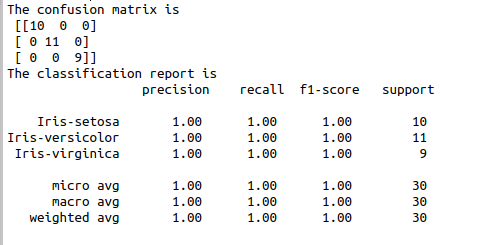• #5, First Floor, 4th Street Dr. Subbarayan Nagar Kodambakkam, Chennai-600 024 Landmark : Samiyar Madam
• pro@slogix.in
• +91- 81240 01111

How to implement KNN algorithm in python?

Description

To implement KNN algorithm using python.

Process

Import library.

Declare X and Y variable.

Split the data into train and test.

Fit the X and Y in to the model.

Make predictions.

Calculate confusion matrix and classification report.

Sapmle Code

#import library functions
import pandas as pd

#took inbuild iris data set
url = (“https://archive.ics.uci.edu/ml/machine-learning-databases/iris/iris.data”)
names = [‘sepal-length’, ‘sepal-width’, ‘petal-length’, ‘petal-width’, ‘Class’]
X = dataset.iloc[:, :-1].values
y = dataset.iloc[:, 4].values

#split the data set
from sklearn.model_selection import train_test_split

#split the sample data in to train and test data
X_train, X_test, y_train, y_test = train_test_split(X, y, test_size=0.20)

#scaling the input train and test data
from sklearn.preprocessing import StandardScaler
scaler = StandardScaler()
scaler.fit(X_train)

#scaling the data
X_train = scaler.transform(X_train)
X_test = scaler.transform(X_test)

#import KNN algorithm from sklearn
from sklearn.neighbors import KNeighborsClassifier
classifier = KNeighborsClassifier(n_neighbors=3)
result=classifier.fit(X_train, y_train)
#print(result)

#make prediction
y_pred = classifier.predict(X_test)
#print the confusion matrix and classification report
from sklearn.metrics import classification_report, confusion_matrix
print(“The confusion matrix is\n”,confusion_matrix(y_test, y_pred))
print(“The classification report is\n”,classification_report(y_test, y_pred))

Screenshots